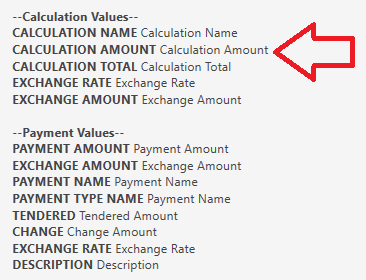# How to get discount percentage

i want to get discount percentage value rather then discount amount. For example total bill is 500 and i give 5% discount when i am printing on template like Discount:|{CALCULATION TOTAL:Discount} it is showing 25 i want percentage that is 5. How can i get this???

If you use a `[DISCOUNTS]` Section, then you can use:

``````{CALCULATION AMOUNT}
``````If you are not using a `[DISCOUNTS]` Section, and you want the value to appear in the main section (`[LAYOUT]` Section), then you will need to derive the percentage using an `[=expression]`, something like:

``````<L00>[=F((TN('{CALCULATION TOTAL:Discount}')/TN('{TICKET TOTAL}'))*100)]%
``````
1 Like

v thanks this solved my problem;